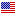1st Edition

# Dichotomies and Stability in Nonautonomous Linear Systems

ISBN 9780415272216
400 Pages
Published October 10, 2002 by CRC PressFree Shipping (6-12 Business Days)
shipping options

• This format is currently out of stock.
ISBN 9780429174926
400 Pages
Published October 10, 2002 by CRC Press

Also available as eBook on:

Linear nonautonomous equations arise as mathematical models in mechanics, chemistry, and biology. The investigation of bounded solutions to systems of differential equations involves some important and challenging problems of perturbation theory for invariant toroidal manifolds. This monograph is a detailed study of the application of Lyapunov functions with variable sign, expressed in quadratic forms, to the solution of this problem. The authors explore the preservation of invariant tori of dynamic systems under perturbation. This volume is a classic contribution to the literature on stability theory and provides a useful source of reference for postgraduates and researchers.

Exponentially Dichotomous Linear Systems of Differential Equations and Lyapunov Functions of Variable Sign. Exponential Dichotomy Criterion for Linear Systems in Terms of Quadratic Forms. Decomposition Over the Whole R Axis of Linear Systems of Differential Equations Exponentially Dichotomous on Semiaxes R+ and R_. Degeneracy of the Quadratic Form Possessing a Definite-Sign Derivative Along the Solutions of the System (1.1.1). Integral Representation of Weakly Regular Systems Bounded on the Whole R Axis. Complement to the Exponentially Dichotomous of Weakly Regular on R Linear Systems. Regularity of Linear Systems of the Block-Triangular Form. Perturbation of the Block-Triangular Form Linear Systems which are Regular and Weakly Regular on the Whole R Axis. Exponentially Dichotomous Linear Systems with Parameters. Comments and References. Linear Extension of Dynamical Systems on a Torus. Necessary Existence Conditions for Invariant Tori. The Green Function. Sufficient Existence Conditions for an Invariant Torus. Existence Conditions for an Exponentially Stable Invariant Torus. Uniqueness Conditions for the Green Function and its Properties. Sufficient Conditions for Exponential Dichotomy of the Invariant Torus. Necessary Conditions for Exponential Dichotomy of the Invariant Torus. Existence Criterion for the Green Function. The Non-Unique Green Function and the Properties of the System Implied by its Existence. Invariant Tori of Linear Extensions with Slowly Changing Phase. Preserving the Green Function Under Small Perturbations of Linear Expansions on a Torus. On the Smoothness of an Exponentially Stable Invariant Torus. On the Dependence of Green Functions on Parameters. Continuity and Differentiability of the Green Function. Invariant Tori of Linear Extensions with a Degenerate Matrix at the Derivatives. Bounded Invariant Manifolds of Dynamical Systems and their Smoothness. Comments and References. Splitability of Linear Extensions of Dynamical Systems on a Torus. Sufficient Conditions for Splitability of Linear Extensions of Dynamical Systems on a Torus. Reversibility of the Theorem on Splitability. On Triangulation and the Relationship of C'-Block Splitability of a Linear System with the Problem on r-frame Complementability up to the Periodic Basis in R^Tn. Reducing on Linearized Systems to a Diagonal Form. On the Relationship of Exponentially Dichotomous Linear Expansions with the Algebraic System Solvability. Three Block Divisibility of Linear Extensions and Lyapunov Functions of Variable Sign. Algebraic Problems of the K-Blocked Divisibility of Linear Extensions on a Torus. Comments and References. Problems of Perturbation Theory of Smooth Invariant Tori of Dynamical Systems. Solution Variations on the Manifold M. Exponential Stability and Dichotomy Conditions for Linear Extensions of Dynamical Systems on a Torus. Roughness Conditions for the Green Function of the Linear Extension of a Dynamical System on a Torus with the Index of Smoothness. A Theorem of Perturbation Theory of an Invariant Torus of a Dynamical System. Green Function for a Linear Matrix Equation. On the Problem of Structure of Some Regular Linear Extensions of Dynamical Systems on a Torus. Invariant Manifolds of Autonomous Differential Equations and Lyapunov Functions with Alternating Signs. Comments and References. Index.
"This volume will be of great interest to researchers and students dealing with nonautonomous systems."
- Zentralblatt fur Mathematik, Vol. 1026

We offer free standard shipping on every order across the globe.